# Area Formula - Definition with Examples

The Complete K-5 Math Learning Program Built for Your Child

• 40 Million Kids

Loved by kids and parent worldwide

• 50,000 Schools

Trusted by teachers across schools

• Comprehensive Curriculum

Aligned to Common Core

## Area Formula

The term “area” refers to the space inside the boundary or perimeter of a closed shape. The geometry of such a shape contains at least three sides joined together to form a boundary. Symbolic representation of such space in mathematics refers to the “area” formula.
To represent and draw real-life objects, designers and architects use different shapes such as circles, triangles, quadrilaterals, and polygons.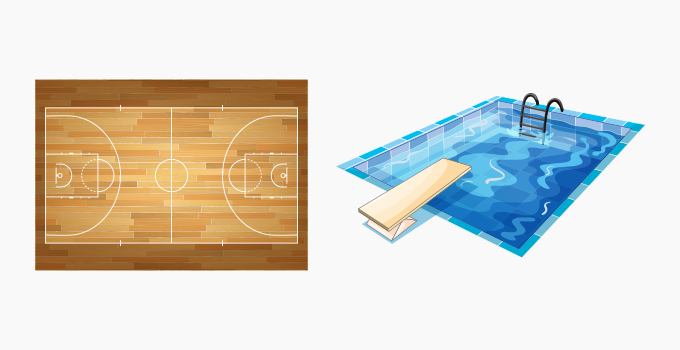Random shapes to show the area covered in a map, of a court and a swimming pool.

The invention of the wheel was the prime step of translating objects into geometric shapes. In the early days, the interpretation of “area” using a formula for geometric shapes evolved from the experiments conducted by Archimedes.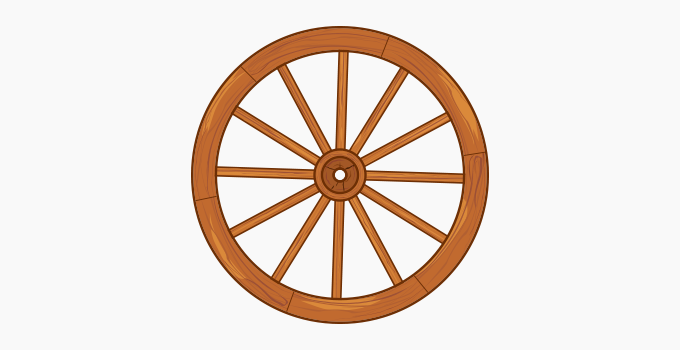## Deriving area formulas

• The “area” for any object  could be  explained as:

• The amount of material (such as paper, fabric, tiles) required to cover the surface in a 2-dimensional plane.

• For a 3-dimensional object such as sphere, ball, cube or cuboid, it is referred to as the surface area.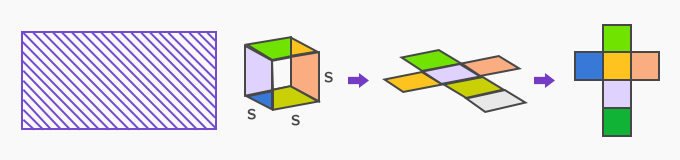## Calculating the area

The easiest method to interpret the area of geometric shapes is using “unit squares”. A unit square is a square with each of its side length measuring 1 unit. Using this as a basis, the area of a polygon is the number of unit squares within a shape.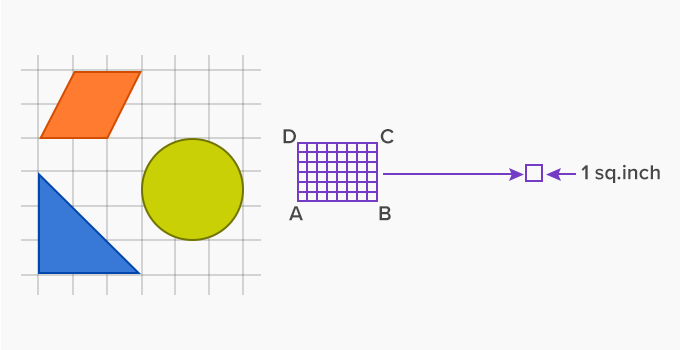The table below lists the shapes and the area formula: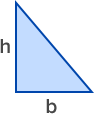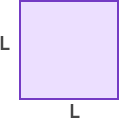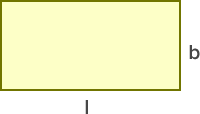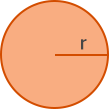Area =  1⁄2× base × height Area = 1⁄2 × b × h Area = length × length Area = l2 Area = length x breadth Area = l × b Area = π × radius × radius Area =  π × r2 (π = 3.14)

Using these formulas, area for different quadrilaterals (a special case of polygons with four sides and angles ≠ 90o) is also calculated.

Application

The concept of area is the foundation of geometry since the early days. Scientists and astronomers took the help of patterns and geometric shapes to understand and establish advanced concepts in science and mathematics. In a modern aspect, the mathematical modeling of objects such as machines, tools, wheels as well as garment designing uses the concept of area and perimeter. It also serves as a basis for integral calculus to understand complex objects such as spheres and ellipses.

 Fun facts  The area of disk was evaluated in the 5th century BCE by Hippocrates of Chios Area of triangle is also calculated using Heron’s formula

Won Numerous Awards & Honors